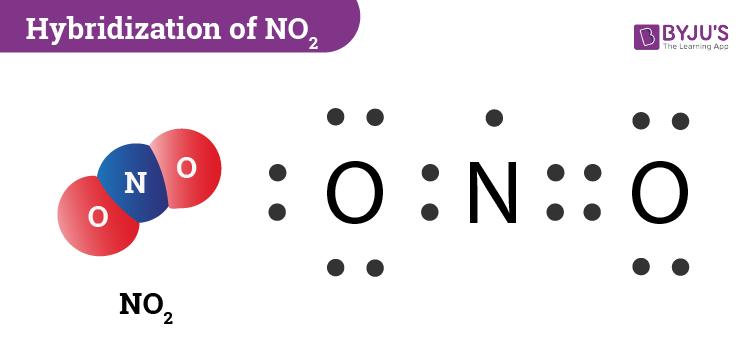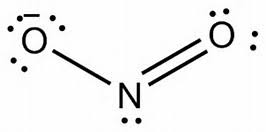# Hybridization of NO2 (Nitrogen Dioxide)

NO2 involves an sp2 type of hybridization. The most simple way to determine the hybridization of NO2 is by drawing the Lewis structure and counting the number of bonds and lone electron pairs around the nitrogen atom. You will find that in nitrogen dioxide there are 2 sigma bonds and 1 lone electron pair.

Now if we apply the hybridization rule then it states that if the sum of the number of sigma bonds, lone pair of electrons and odd electrons is equal to three then the hybridization is sp2. We will discuss this topic in detail below.

 Name of the Molecule Nitrogen Dioxide Molecular Formula NO2 Hybridization Type sp2 Bond Angle 134o Geometry Bent

## What is the Hybridization of Nitrogen Dioxide?

During the formation of NO2, we first take a look at the Nitrogen atom. Here you will notice that the nitrogen atom is the centre atom and has one lone electron. However, this atom does not have an octet as it is short on electrons. Since there is a deficit of electron in the nitrogen molecule it usually tends to react with some other molecule (in this case oxygen) to complete its octet. The two oxygen atoms, on the other hand, have an octet of electrons each. When the bonding takes place, the two atoms of oxygen will form a single and a double bond with the nitrogen atom.Meanwhile, nitrogen must have three hybridized orbitals that will be used to harbour two sigma bonds and one electron. This results in sp2 hybridization. The three sp2 hybrid orbitals in nitrogen will contain one electron and the p orbital will also contain one electron. However, when it forms the two sigma bonds only one sp2 hybrid orbital and p orbital will contain one electron each. The p orbital will form a pi bond with the oxygen atom.

### Important Points To Remember

• In nitrogen dioxide, there are 2 sigma bonds and 1 lone electron pair.
• The two oxygen atoms have an octet of electrons each.
• The p orbital of nitrogen forms a pi bond with the oxygen atom.

## NO2 Molecular Geometry And Bond Angles

Since NO2 has an extra electron in an orbital on the nitrogen atom it will result in a higher degree of repulsions. However, if we take the one lone electron or the single-electron region there is less repulsion on the two bonding oxygen atoms. So the repulsions are not identical. As a result, the oxygen atoms are spread widely.The bond angle is 134o which is actually far from the ideal angle of 120o. NO2 molecular geometry will be bent.